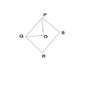# Geometry Question

physics kiddy
Recently, I bought a book and found a strange question :

In the given figure, PQRS is a quadrilateral. PO and QO are bisectors of angle P and angle Q respectively, then prove that angle QOP = 1/2 (angle R + angle S)

I made several attempts to get the solution but failed. I guess, we need to prove that PQRS is a parallelogram.

#### Attachments

•Question.jpg
4.5 KB · Views: 346

physics kiddy
It's 540 degrees.

Homework Helper
It's 540 degrees.

OK. How is the angle a related to p/2 and q/2? What is the sum of angles of the pentagon in terms of a, p/2, q/2, r and s?

ehild

Last edited:
Staff Emeritus
In triangle QOP, determine angle QOP, hence determine reflex angle QOP.

Homework Helper
Physics kiddy disappeared...

p/2+q/2=180-a (triangle)
p/2+q/2+r+s+360-a=540 (pentagon) -->

180-a+r+s+360-a=540, 2a=r+s.

ehild

physics kiddy
Thank you ! Thank you again. I disappeared because I found the solution in a book. But you wrote a completely new and easy method to solve this question. Now, I have got two ways to solve this question.

Homework Helper
I would like to see that solution from the book so much! Could you please show it?ehild